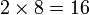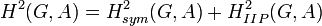# Second cohomology group for trivial group action of direct product of Z4 and Z2 on Z2

This article gives information about the second cohomology group for trivial group action (i.e., the second cohomology group with trivial action) of the group direct product of Z4 and Z2 on cyclic group:Z2. The elements of this classify the group extensions with cyclic group:Z2 in the center and direct product of Z4 and Z2 the corresponding quotient group. Specifically, these are precisely the central extensions with the given base group and acting group.
The value of this cohomology group is elementary abelian group:E8.
Get more specific information about direct product of Z4 and Z2 |Get more specific information about cyclic group:Z2|View other constructions whose value is elementary abelian group:E8

## Description of the group

We consider here the second cohomology group for trivial group action of the direct product of Z4 and Z2 on the cyclic group:Z2, i.e.,$\! H^2(G,A)$

where$G \cong \mathbb{Z}_4 \times \mathbb{Z}_2$ and$A \cong \mathbb{Z}_2$.

The cohomology group is isomorphic to elementary abelian group:E8.

## Computation of cohomology group

The cohomology group can be computed as an abstract group using the group cohomology of direct product of Z4 and Z2.

The general formula for$H^2(G;A)$ for this choice of$G$ is:$A/4A \oplus A/2A \oplus \operatorname{Ann}_A(2)$

In this case, with$A = \mathbb{Z}/2\mathbb{Z}$, all three summands are$\mathbb{Z}/2\mathbb{Z}$, so overall we get$(\mathbb{Z}/2\mathbb{Z})^3$.

## Elements

FACTS TO CHECK AGAINST (second cohomology group for trivial group action):
Background reading on relationship with extension groups: Group extension problem
Arithmetic functions of extension group:
order (thus all extension groups have the same order): order of extension group is product of order of normal subgroup and quotient group
nilpotency class: nilpotency class of extension group is between nilpotency class of quotient group and one more for central extension
derived length: derived length of extension group is bounded by sum of derived length of normal subgroup and quotient group
minimum size of generating set: minimum size of generating set of extension group is bounded by sum of minimum size of generating set of normal subgroup and quotient group|minimum size of generating set of quotient group is at most minimum size of generating set of group
WHAT'S THE TABLE BELOW?: Recall that there is a correspondence:
Elements of the group$H^2(G;A)$ for the trivial group action$\leftrightarrow$ congruence classes of central extensions with the specified subgroup$A$ and quotient group$G$.
This descends to a correspondence:
Orbits for the group action of$\operatorname{Aut}(G) \times \operatorname{Aut}(A)$ on$H^2(G;A)$$\leftrightarrow$ pseudo-congruence classes of central extensions.
The table below breaks down the second cohomology group as a union of these orbits, with (as a general rule) each row describing one orbit, i.e., one "cohomology class type", aka one "pseudo-congruence class" of central extensions. The number of rows is the number of pseudo-congruence classes of central extensions.

We list here the elements, grouped by similarity under the action of the automorphism groups on both sides.

In all cases, order of extension group is product of order of normal subgroup and quotient group, so the order is$2 \times 8 = 16$. Also, since these are central extensions (because the action is trivial), the nilpotency class of the extension group is at least 1 (the nilpotency class of the quotient) and at most 2.

The minimum size of generating set is at least 2 (the minimum size of generating set for the quotient) and at most 3 (the sum of the minimum size of generating set for the normal subgroup and the quotient). See minimum size of generating set of extension group is bounded by sum of minimum size of generating set of normal subgroup and quotient group and minimum size of generating set of quotient group is at most minimum size of generating set of group.

The Schur multiplier$H_2(G;\mathbb{Z})$ is cyclic group:Z2, which is the base of the extension. Thus, some of these extensions are stem extensions, and the corresponding extension groups are Schur covering groups for$G$.

Cohomology class type Number of cohomology classes Corresponding group extension Second part of GAP ID (order is 16) Stem extension? Base characteristic in whole group? Hall-Senior family (equivalence class up to being isoclinic) Nilpotency class of whole group (at least 1, at most 2) Derived length of whole group (at least 1, at most 2) Minimum size of generating set of whole group (at least 2, at most 3) Subgroup information on base in whole group
trivial 1 direct product of Z4 and V4 10 No No$\Gamma_1$ 1 1 3
symmetric and nontrivial 2 direct product of Z8 and Z2 5 No Yes$\Gamma_1$ 1 1 2
symmetric and nontrivial 1 direct product of Z4 and Z4 2 No No$\Gamma_1$ 1 1 2
non-symmetric 1 SmallGroup(16,3) 3 Yes Yes$\Gamma_2$ 2 2 2 derived subgroup of SmallGroup(16,3)
non-symmetric 2 M16 6 Yes Yes$\Gamma_2$ 2 2 2 derived subgroup of M16
non-symmetric 1 nontrivial semidirect product of Z4 and Z4 4 Yes Yes$\Gamma_2$ 2 2 2 derived subgroup of nontrivial semidirect product of Z4 and Z4
Total (6 types) 8 -- -- -- --$\Gamma_1, \Gamma_2$ -- -- -- --

## Group actions

### Under the action of the automorphism group of the acting group

Under the action of$\operatorname{Aut}(G)$, there are six orbits -- one for each of the cohomology class types listed above. In particular, there are four fixed points: direct product of Z4 and V4, direct product of Z4 and Z4, SmallGroup(16,3), and nontrivial semidirect product of Z4 and Z4. There are two orbits of size two: direct product of Z8 and Z2 and M16.

## Subgroups of interest

Subgroup Value Corresponding group extensions for subgroup GAP IDs second part Group extension groupings for each coset GAP IDs second part
IIP subgroup of second cohomology group for trivial group action cyclic group:Z2 direct product of Z4 and V4, SmallGroup(16,3) 10, 3 (direct product of Z4 and V4, SmallGroup(16,3)) (1 copy), (direct product of Z8 and Z2, M16) (2 copies), (direct product of Z4 and Z4, nontrivial semidirect product of Z4 and Z4) (1 copy) (10, 3) (1 copy), (5, 6) (2 copies), (2, 4) (1 copy)
cyclicity-preserving subgroup of second cohomology group for trivial group action trivial group direct product of Z4 and V4 10 each element is its own coset 10, 5, 2, 3, 6, 4
subgroup of second cohomology group comprising symmetric 2-cocycles, corresponds to abelian group extensions Klein four-group direct product of Z4 and V4 (1 copy), direct product of Z8 and Z2 (2 copies), direct product of Z4 and Z4 (1 copy) 10, 5, 2 (direct product of Z4 and V4 (1 copy), direct product of Z8 and Z2 (2 copies), direct product of Z4 and Z4 (1 copy)) (1 copy), (SmallGroup(16,3) (1 copy), M16 (2 copies), nontrivial semidirect product of Z4 and Z4 (1 copy)) (1 copy) (10, 5, 2) and (3, 6, 4)

## Direct sum decomposition

For background information, see formula for second cohomology group for trivial group action of abelian group in terms of Schur multiplier and abelianization

### General background

We know from the general theory that there is a natural short exact sequence:$0 \to \operatorname{Ext}^1_{\mathbb{Z}}(G,A) \to H^2(G;A) \stackrel{\operatorname{Skew}}{\to} \operatorname{Hom}(\bigwedge^2G,A) \to 0$

where the image of$\operatorname{Ext}^1$ is$H^2_{sym}(G;A)$, i.e., the group of cohomology classes represented by symmetric 2-cocycles. We also know, again from the general theory, that the short exact sequence above splits, i.e.,$H^2_{sym}(G;A)$ has a complement inside$H^2$. However, there need not in general be a natural or even an automorphism-invariant choice of splitting.

### In this case

For this choice of$G$ and$A$, the subgroup$H^2_{CP}(G;A)$ of cyclicity-preserving cohomology classes is trivial, hence the sum$H^2_{sym}(G;A) + H^2_{CP}(G;A)$ is$H^2_{sym}(G;A)$ and is not the whole group$H^2(G;A)$. Thus,$H^2_{CP}(G;A)$ is not the desired complement.

However, the subgroup$H^2_{IIP}(G,A)$ (the IIP subgroup of second cohomology group for trivial group action) is a complement to$H^2_{sym}(G,A)$ in$H^2(G,A)$. This comprises the trivial extension and the extension that gives SmallGroup(16,3). Further, this complement is invariant under the action of$\operatorname{Aut}(G) \times \operatorname{Aut}(A)$. As an internal direct sum:$H^2(G,A) = H^2_{sym}(G,A) + H^2_{IIP}(G,A)$

as an internal direct sum. A pictorial description of this would be as follows. Here, each column is a coset of$H^2_{IIP}(G,A)$ and each row is a coset of$H^2_{sym}(G,A)$. The top left entry is the identity element, hence the top row corresponds to abelian group extensions and the left column corresponds to$H^2_{IIP}(G,A)$.

The group$\operatorname{Aut}(G) \times \operatorname{Aut}(A)$ acts to permute the two right columns. Both rows remain intact. The two left columns also remain intact.

## GAP implementation

### Construction of the cohomology group

The cohomology group can be constucted using the GAP functions CyclicGroup, DirectProduct, TwoCohomology, TrivialGModule, GF.

gap> G := DirectProduct(CyclicGroup(4),CyclicGroup(2));;
gap> A := TrivialGModule(G,GF(2));;
gap> T := TwoCohomology(G,A);
rec( group := <pc group of size 8 with 3 generators>, module := rec( field := GF(2), isMTXModule := true, dimension := 1,
generators := [ <an immutable 1x1 matrix over GF2>, <an immutable 1x1 matrix over GF2>, <an immutable 1x1 matrix over GF2> ] ),
collector := rec( relators := [ [ [ 2, 1 ] ], [ [ 2, 1 ], 0 ], [ [ 3, 1 ], [ 3, 1 ], 0 ] ], orders := [ 2, 2, 2 ], wstack := [ [ 2, 1 ], [ 2, 1 ] ],
estack := [  ], pstack := [ 3, 3 ], cstack := [ 1, 1 ], mstack := [ 0, 0 ], list := [ 1, 1, 0 ],
module := [ <an immutable 1x1 matrix over GF2>, <an immutable 1x1 matrix over GF2>, <an immutable 1x1 matrix over GF2> ],
mone := <an immutable 1x1 matrix over GF2>, mzero := <an immutable 1x1 matrix over GF2>, avoid := [  ], unavoidable := [ 1, 2, 3, 4, 5, 6 ] ),
cohom := <linear mapping by matrix, <vector space of dimension 4 over GF(2)> -> ( GF(2)^3 )>,
presentation := rec( group := <free group on the generators [ f1, f2, f3 ]>, relators := [ f1^2*f2^-1, f1^-1*f2*f1*f2^-1, f2^2, f1^-1*f3*f1*f3^-1,
f2^-1*f3*f2*f3^-1, f3^2 ] ) )

### Construction of extensions

The extensions can be constructed using the additional command Extensions.

gap> G := DirectProduct(CyclicGroup(4),CyclicGroup(2));;
gap> A := TrivialGModule(G,GF(2));;
gap> L := Extensions(G,A);;
gap> List(L,IdGroup);
[ [ 16, 10 ], [ 16, 2 ], [ 16, 3 ], [ 16, 4 ], [ 16, 5 ], [ 16, 5 ], [ 16, 6 ], [ 16, 6 ] ]

### Under the action of the various automorphism groups

This uses additionally the GAP functions AutomorphismGroup, DirectProduct, CompatiblePairs, and ExtensionRepresentatives.

gap> G := DirectProduct(CyclicGroup(4),CyclicGroup(2));;
gap> A := TrivialGModule(G,GF(2));;
gap> A1 := AutomorphismGroup(G);;
gap> A2 := GL(1,2);;
gap> D := DirectProduct(A1,A2);;
gap> P := CompatiblePairs(G,A,D);;
gap> M := ExtensionRepresentatives(G,A,P);;
gap> List(M,IdGroup);
[ [ 16, 10 ], [ 16, 2 ], [ 16, 3 ], [ 16, 4 ], [ 16, 5 ], [ 16, 6 ] ]